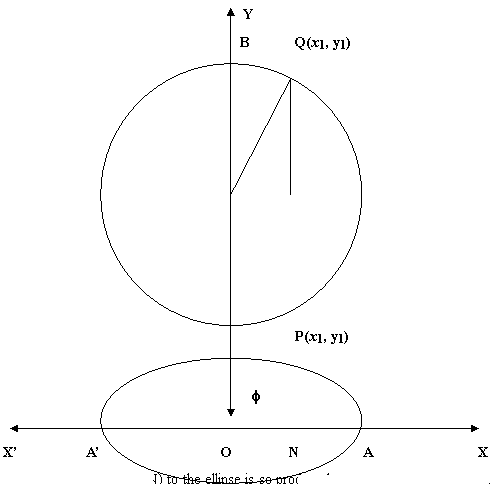# Math Assignment Help With Eccentric angle

## 7.6.7 Eccentric angle:

###When a perpendicular(PN) to the ellipse is so produced that it touches the auxiliary circle at some point (Q), then the angle thus produced ( NOQ) is called the Eccentric angle.

The ordinates of any point P on an ellipse and its corresponding point Q on its auxiliary circle are in constant ratio. i.e.

y1 ‗ b ‗ a √(1 – e2) = √(1 – e2)

y2 a a